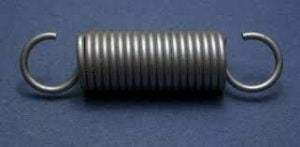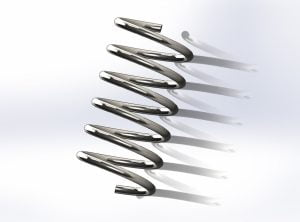Monday, October 2, 2023

# Helical Spring Numericals

### 1. Introduction – Spring

Springs are elastic members which distort under load and retain their original shape when they are removed.

They are used in railway carriers, motor cars, scooters, motorcycles, rickshaws, etc.

### 2. Helical Spring

A helical spring is a length of wire or bar wound into a helix.

It may be of two types:

1. Close Coiled Helical Spring2. Open Coiled Helical Spring### 3. Formulas For Helical Spring

Let us consider,

d= Diameter of wire of the coil

δ= deflection of the coil under load W

C= Modulus of rigidity

n= Number of coils or turns

θ= angle of twist

l= length of wire =2πRn

τ = Shear Force

Ip = Polar moment of inertia = πd4 / 32

a. From Torsional relation,

 T= (π τ d3 ) / 16

 τ = ( 16WR ) / πd3

b. Deflection of Coil (δ)

 δ= ( 8 WD3  n ) / ( Cd4   )  or δ = R x θ

 θ= ( 16 WD2  n ) / ( Cd4  )

c. Stiffness or, Spring Constant (K)

 K= ( Cd4  ) / ( 8 D3  n )   or K = W/δ

d. Weight of Spring (W)

W = K x δ

e. WAHL’s Factor

 K= ( HC-1/ HC-4 )      +     ( 0.615 / C )

where,

C= Spring Index = D / d

f. Solid Length of Spring

Solid Length ( L) = n’ x d

where,

n’ = total number of active coils

### 4. Numericals

#### a. For Closely Coiled Helical Spring

1. A closely coiled helical spring is used to carry a load of 500 N. Its mean coiled diameter is to be 10 times that of wire diameter. Calculate the diameters if the maximum shear stress in the material of the spring is to be 80 MN/m2  .

Given,

Mean coiled diameter (D)= 10X Wire diameter

or, 2R=10d

∴ R=5d m

τ (max shear stress ) = 80 MN/m² = 80 x 106 N/m²

we know,

τ = (16WR) / πd3

or, 80x 106  = 16x500x5d / πd3

or, d²  = (16x500x5) / π x 80 x 106

or,  d = 0.0126 m

∴ d= 12.6 mm

∴ D= 2R = 2 x 5d = 10 x 12.6 = 126mm

2. A helical spring is made of a 12 mm diameter steel wire wound on a 120 mm diameter mandrel. If there are 10 active coils, what is spring constant? Take C=82 GN/m2. What force must be applied to the spring to elongate it by 40 mm?

Given,

Diameter of wire (d) = 12 mm

Diameter of Coil (D=2R) = 120mm

Deflection (δ)= 40 mm

R= 60 mm

n = 10

K=?

C= 82 GN/m2  = 82000 N/mm2

We know,

Spring Constant (K) = ( Cd ) / ( 64 R3  n )

K = (82000×124 )/ (64x 603 x 10)

K = 12.3 N/mm

Again,

K= W / δ

W= 40 x 12.3 = 492N

W= 492N is the required force to be applied to the spring.

#### b. For Open Coiled Helical Spring

3. An Open coiled helical spring made from wire of circular cross-section is required to carry the load of 120 N. The wire diameter is 8 mm and the means coil radius is 48 mm. If the helix angle of the spring is 30° and the number of turns is 12. Calculate,

i. Axial Deflection

ii. Angular rotation of free end with the respect to the fixed end of the spring.

Take C= 80 GN/m2  ,  E= 200 GN/m2

Solution,

Diameter of wire (d)= 8 mm = 0.008 m

Mean radius of coil (R)= 48 mm =0.048 m

Helix angle (α)= 30°

Number of turns (n)= 12

Modulus of rigidity (C)= 80 GN/m2

Young’s Modulus (E)= 200 GN/m2

(i) Axial deflection δ:

Axial deflection is given by,

δ = 2W R3 n π sec α [(Cos2 α / CIp)  +  (Sin2 α / EI)]

δ = 2x 120x (0.048)2 x 12 x π x Sec 30° [Cos2 α / {80×109 x π/32 x (0.008)4 }]  +  [Sin2 α /{200 x 109 x π/32 x (0.008)4)}]

δ = 34.1 mm

Hence,

Angular Rotation,

φ = 2WR2 n π sin α [ (1/CIp) – (1/EI) ]

φ = 2 x 120 x (0.048)2 x 12 x π x Sin30° [ (1/ {80×109 x π/32 x (0.008)4 } – 1/ {80×109 x π/32 x (0.008)4 }]

φ =3.71°

4. An open coiled helical spring of wire diameter 12 mm, mean coil radius 84 mm, helix angle 20° carries an axial load of 480 N. Determines the shear stress and direct stress developed at the inner radius of the coil.

Given,

Diameter of wire (d)= 12 mm = 0.012m

Mean coil radius (R)= 84 mm = 0.084m

Helix angle (α)= 20°

Shear stress

Twisting Moment, T= WR cosα

Bending Moment, M= WR sinα

Torsional shear stress, (τ 1) = 16T/ πd3

= (16x 480x 0.084 x cos20°)/ (π x 0.0123 )            ( T= WR cosα )

= 111.06 MN/m2

Direct Shear Stress (τ 2) = 4W/ πd2

= (4 x480) / π 0.0122

= 4.24 MN/m2

Total Shear stress at the inner coil radius,

τ = τ1 + τ2

τ = 115.9 MN/m2

Direct stress due to bending,

σ = (32 M) / (π d³)

σ = (32 x W R sinα) / π d3

σ = (32x 480 x 0.084x sin 20°x 10-6 ) / (π (0.012)³)

σ = 81.28 MN/m2

 Read Also: Rise and Fall Method
 Read Also: Numerical of Theodolite TraversingHe is a founder and lead author of Dream Civil International and his civil engineering research articles has been taken as source by world's top news and educational sites like USA Today, Time, The richest, Wikipedia, etc.
Latest Articles

Related Articles